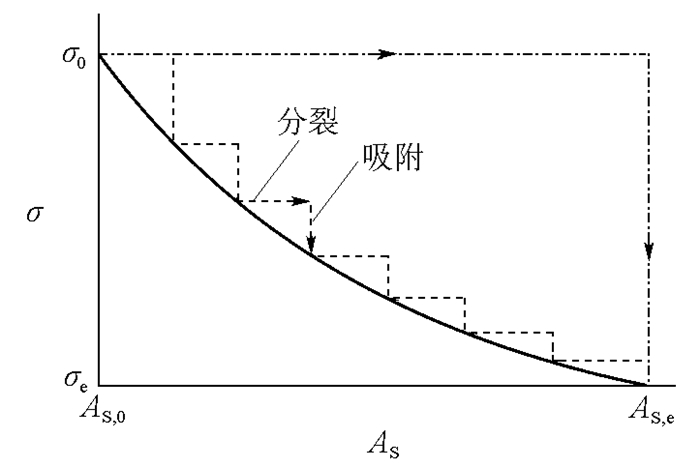## The Additional Pressure and the Stability of Disperse System

,,

 基金资助: 华东理工大学网络教育教改项目.  WJY2014006国家精品资源共享课建设项目.  YJ0125206

 Fund supported: 华东理工大学网络教育教改项目.  WJY2014006国家精品资源共享课建设项目.  YJ0125206Abstract

We discussed the relationship between the additional pressure and the stability of disperse system in detail.It was found that the reduction of additional pressure of disperse phase makes the disperse system become more stable.Taking the micro-emulsion as an illustration, the reduction of additional pressure lowers the collision probability among liquid droplets, and more importantly, it is closely associated with the formation of a strong protective film on the droplets.The disperse system could reach the thermodynamic stability state only when the additional pressure of the droplet tends to become zero, and the emulsion becomes micro-emulsion.The above discussion could also be applied to the solid/liquid disperse systems.

Keywords： Additional pressure ; Disperse system ; Specific surface entropy ; Interface internal pressure ; Micro-emulsion

LIU Guo-Jie, HEI En-Cheng. The Additional Pressure and the Stability of Disperse System. University Chemistry[J], 2016, 31(1): 54-58 doi:10.3866/pku.DXHX20160154

## 1 附加压力与化学势

$\quad \quad \quad \quad \Delta p = \frac{{2\sigma }}{r}$

$\quad \quad \quad \quad {\left( {\frac{{\partial \mu }}{{\partial p}}} \right)_T} = {V_{\scriptsize{\rm{m}}}}$

## 2 分散相的附加压力与界面内压力

$\quad \quad \quad \quad G = H - TS$

$\quad \quad \quad \quad \sigma = {H_{{A \! _{\rm{s}}}}} - T{S_{{A \! _{\rm{{s}}}}}}$

$\quad \quad \quad \quad {\sigma _{\rm{e}}}{A \! _{{\rm{s,e}}}} = {\sigma _0}{A \!_{{\rm{s}},0}}$

$\quad \quad \quad \quad {\sigma _{\rm{e}}} = \frac{{{A \! _{{\rm{s}},0}}}}{{{A \! _{{\rm{s}},{\rm{e}}}}}}{\sigma _0}$

### 图3$\quad \quad \quad \quad {\sigma _{\rm{e}}} \ll {\sigma _0}$

$\quad \quad \quad \quad \Delta p = \frac{{2{\sigma _{\rm{e}}}}}{{{r_{\rm{e}}}}} \to 0$

## 参考文献 原文顺序 文献年度倒序 文中引用次数倒序 被引期刊影响因子

/

 〈〉